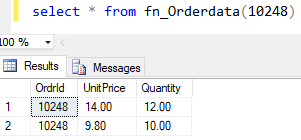Home » USER DEFINED FUNCTIONS

# USER DEFINED FUNCTIONSSQL Server allows us to create our functions called as user defined functions in SQL Server.

User defined functions is reusable, Instead of writing same logic again and again, you can create a user defined function of it.

There are two types of SQL user defined functions as given below.

1. Scalar Function: This function always returns a single scalar data value. BEGIN .. END block is used in Scalar function.
2. Table Valued Functions: It is a user defined function in SQL Server that returns a tabular result set. There are two types of Table Valued functions as given below.
• Inline Table valued Functions: This function returns a tabular result set based on a single Select Statement .
• Multi-Statement Table valued Functions: This function also returns the tabular result set. But, unlike the inline table valued function, It consists a series of T-SQL statements inside the function body and it has a BEGIN.. END block.

#### Scalar Function

SYNTAX

```CREATE FUNCTION fn_name (@parameter1 datatype1, @parameter2 datatype2,  ...)
RETURNS  datatype
AS BEGIN
RETURN
{expression }
END```

#### To get the sum of values using scalar function

Lets, create a User defined scalar functions named Fn_CalulateSum that returns the sum of two input values.

```CREATE FUNCTION Fn_CalulateSum( @A Int , @B Int)
RETURNS  INT
AS  BEGIN
RETURN (@A + @B)
END```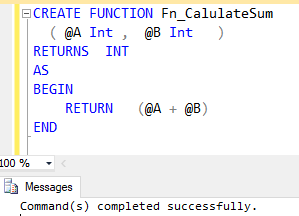As you can see, the output of function for input parameter 10 & 125, it returns the sum of parameter values 10 and 125 that is = 135.

`Select dbo.Fn_CalulateSum (10 ,125) as Get_Sum`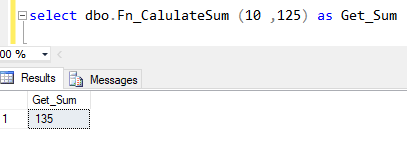Lets, look at another example of creating a scalar function.

#### To get the sum of unit price for a specific order Id using scalar function

Following scalar function named Fn_getOrderPrice, returns the total sum of unit price based on input value for OrderId.

```CREATE FUNCTION Fn_getOrderPrice( @OrderId Int )
RETURNS  INT
AS BEGIN
RETURN
(
Select sum(UnitPrice) as totalprice
From [Order Details] Where orderId = @OrderId
)
END```

As you can see the function output, it returns the total Unitprice for order Id = 10248

`select dbo.Fn_getOrderPrice (10248)as total_unitPrice`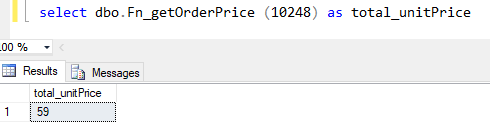#### Inline Table Valued Function

SYNTAX

```CREATE FUNCTION fn_name (
@parameter1 datatype1, @parameter2 datatype2, ... )
RETURNS TABLE AS RETURN(select .......)```

#### To get the Order details for a specific order Id using Inline table valued function

Following Inline table valued functions named Fn_getOrderDetails, returns the order details for provided order id value.

```CREATE FUNCTION Fn_getOrderDetails (@OrderId Int)
RETURNS TABLE  AS  RETURN
Select * from [Order Details] where orderId = @OrderId```Here is the output of function, it returns the order details for order Id =10248.

`Select * from Fn_getOrderDetails(10248)`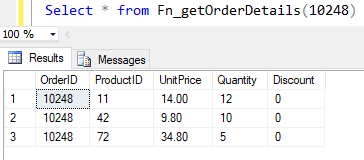#### Multi-Statement Table Valued Function

SYNTAX

```CREATE FUNCTION fn_name( @parameter1  datatype1, @parameter2  datatype2 , ... )
RETURNS @returntablename TABLE (column1 datatype1, column2 datatype2,.....)
AS BEGIN
INSERT INTO @returntablename SELECT.....
RETURN
END```

Following Multi-statement Table Valued function returns OrderId, Unitprice and Quantity from table [Order details] for a specific order id also deletes the records from output for which quantity value is <=5.

```CREATE FUNCTION fn_Orderdata(@OrderId Int)
RETURNS @ordrtbl
TABLE(OrdrId int, UnitPrice numeric(18,2), Quantity numeric(18,2))
AS BEGIN
INSERT INTO @ordrtbl SELECT orderId, unitPrice, quantity
from [Order Details]
WHERE orderId =@OrderId
Delete from @ordrtbl where Quantity <=5;
RETURN
END```Here, is the output of Multi-statement table valued function for Order Id =1028, also you can see, there is no quantity which is less then equal to 5.

`select * from fn_Orderdata (10248)`